×#### Thank you for registering.

One of our academic counsellors will contact you within 1 working day.

Click to Chat

1800-1023-196

+91-120-4616500

CART 0

• 0

MY CART (5)

Use Coupon: CART20 and get 20% off on all online Study Material

ITEM
DETAILS
MRP
DISCOUNT
FINAL PRICE
Total Price: Rs.

There are no items in this cart.
Continue Shopping```Solution of System of Linear Inequalities in Two Variables

Table of Content

Meaning of System of Linear Inequality

Solution of System of Linear Inequalities in Two Variables

Graphical Method

Non-Graphical Method

Difference between Graphical and Non-Graphical Method

Word Problem

Meaning of System of Linear Inequality

A System of Linear Inequalities is the set of two or more linear inequalities which are having same variables.

Example

x + y ≥ 5

x – y ≤ 3

Here are two inequalities having two same variables that is, x and y.

Solution of System of Linear Inequalities in Two Variables

The solution of a system of linear inequality is the ordered pair which is the solution of all inequalities in the considered system and the graph of the system of linear inequality is the graph of common solution of the system.

So the Solution of the System of Linear Inequalities could be:

Graphical

Non-graphical

Graphical Method

Graphical Method for the Solution of the System of Linear Inequalities is the easiest method. In this method the process of making a graph is similar to the graph of linear inequalities in two variables.

Steps of Solution of System of Linear Inequalities in Two Variables

First of all plot all the given lines of inequalities on the same Cartesian plane, in the system of linear inequalities it could be two or more lines of inequalities.

Make the line dotted for < or > sign, and a solid line for ≤ or ≥ sign.

Now shade the solution region according to the sign of inequality that is, below the line for < or ≤ sign and above the line for > or ≥ sign.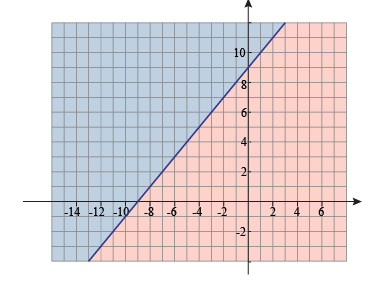As here blue region is above the line and pink region is below the line.

Now the solution of the system of inequalities is the shaded region which is common in the solution of all the line of inequalities.

The solution could be

open or unbounded

closed or bounded

no solution

Example:1

Solve the system of inequalities graphically.

Y > -3x +5

Y ≤ x-2

Solution:

First we will draw both the inequalities on the same Cartesian plane.

Draw a dotted line for the first inequality as it is a strict inequality and shade the region above the line.

Draw a solid line for the second inequality as it is a slack inequality and shade the region below it.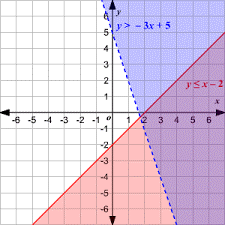The solution of this system of inequality is the intersected shaded region or the shaded region which is common to both the inequalities.

Let’s check our solution

It shows that any point (3,-1) is the solution of the system of inequalities according to the intersected shaded region.

Y > -3x +5
Y ≤ x-2

-1 > - 3 (3) +5
-1 ≤ 3-2

TRUE
-1 ≤ 1

TRUE

TRUE
True

Hence (3,-1) is the solution of the system of inequality.

This is the open solution as it is unlimited at the one end or it is not bounded by the lines from all sides.

Example: 2

Solve the system of inequalities graphically.

x≤ 8

y≥ 2

2y-x ≤ 6

Solution:

This is the system of inequalities with three inequalities.

First we will solve the third inequality for y.

2y-x ≤ 6

2y ≤ x+6

Now we will make all the three lines of inequalities solid as these are slack inequalities.

Shade the region according to the sign of inequalities.

Now the shaded region common in all the inequalities is the solution of system of inequalities.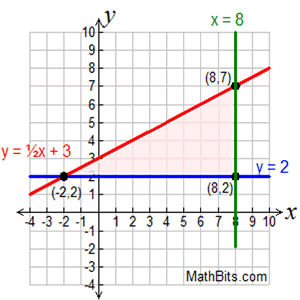It is the closed solution as three lines bound it.

Let’s check any point (2, 3) for the solution of the system of inequalities.

x ≤ 8
y ≥ 2
2y-x ≤ 6

2 ≤ 8
3 ≥ 2
2(3) – 2 ≤ 6

6-2 ≤ 6

4 ≤ 6

TRUE
TRUE
TRUE

Hence this is the solution of the system of inequalities as all the point in the common shaded region is the solution of the system of linear inequalities.

Example: 3

Solve the system of inequalities graphically.

y ≤ -3-x

y ≥ 3-x

Solution:

Here we don’t have to solve for y as the inequalities already have y on the left side.

So we will draw the solid line for both the inequalities on the same Cartesian plane as both are the non-strict inequalities.

Shade the solution region of both the inequalities according to their sign of inequality.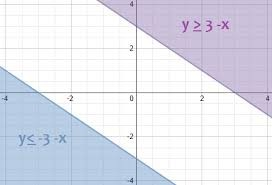Here we can see that there is no common region in both the inequalities.

So, here is no solution of the system of inequalities. The two inequalities are mutually exclusive inequalities.

Non-Graphical Method

In the Non-Graphical Method, there is no need to make a graph but we can find the solution of the system of inequalities by finding the interval at which the systems satisfy all the inequalities.

In this method, we have to find the point of intersection of the two inequalities by solving them. It could be possible that there is no intersection point between them. If there is no intersection point then

It could be mutually exclusive inequalities as we have seen above in the graphical method, the inequalities with no solution i.e. no common shaded region.

One inequality is the subset of the other inequality. If all the solutions of first inequality are the solutions of second inequality also then the second inequality is the subset of the first one. Lets understand it with the graph of two inequalities.This shows that the solution of the first inequality is the solution of the second inequality also . so the first inequality is the subset of the second inequality.

Example

Solve the inequalities with non-graphical method.

y ≤ x-2

y > -3x +5

Solution:

We will find the solution i.e. the intersection point of the above two inequalities by substituting y = x-2 in second inequality.

y > -3x +5

x – 2 > -3x + 5 (by substituting y as x-2)

x + 3x > 5 + 2

4x > 7

Now we have the solution as x > 7/4 or 1.75

So, we will check it by assuming x as anything more than 1.75

Let x = 2

Substitute x = 2 in both the given inequalities.

y ≤ x - 2
y > – 3x + 5

y ≤ 2 – 2
y > – 3(2) + 5

y ≤ 0
y > – 1

This shows that the two inequalities are not mutually exclusive, so they will satisfy for x > 7/4

Difference between Graphical and Non-Graphical Method

Graphical method is the easiest way to find the solution of the system of linear inequalities compared to which non-graphical method is difficult.

We can get all the solutions in the graphical method but in non-graphical method it is not possible to visualize all the solutions in case of more variables.

In case of more inequalities, graphical method is the feasible method instead of non-graphical method as it is not possible to solve more than two inequalities with the non graphical method.

Word Problem

Sandra is selling jewellery and stationery for 4\$ and 5\$ respectively. She wants to make 75\$ daily by her business. But she can buy only 15 items altogether in a day. Solve this by both the methods.

Solution:

First of all we have to write the inequalities according to the problem.

Let no. of jewellery = x and no. of stationery y.

4x + 5y ≤ 75

x + y ≤ 15

Graphical Method

First we will draw the line of inequalities as solid line because both are the non-strict inequalities.

Then shade the solution region with the different colors.

Now the common shaded region is the solution region of the system of inequalities.

We can take any ordered pair from the intersected shaded region to check the solution of the system.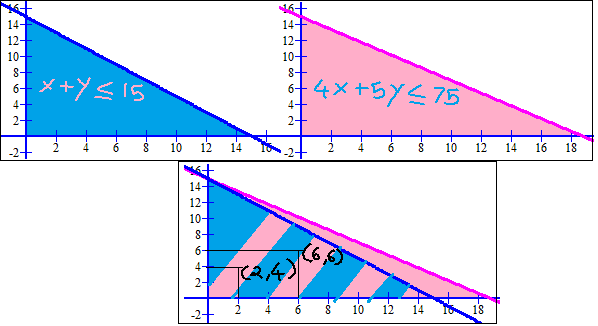Let’s check for (2, 4) and (6, 6)

(2,4)

4x + 5y ≤ 75
x + y ≤ 15

4(2) + 5(4) ≤ 75
2+4 ≤ 15

8 + 20 ≤ 75
6 ≤ 15

28 ≤ 75

TRUE
TRUE

Hence (2, 4) is the solution of the system of inequality.

(6,6)

4x + 5y ≤ 75
x + y ≤ 15

4(6) + 5(6) ≤ 75
6+6 ≤ 15

24 + 30 ≤ 75
12 ≤ 15

54 ≤ 75

TRUE
TRUE

Hence (6, 6) is also the solution of the system of inequality.

Non Graphical Method

4x + 5y ≤ 75

x + y ≤ 15

We will solve the second inequality for y.

x + y ≤ 15

y ≤ 15 –x

Substitute y in the first inequality.

4x + 5y ≤ 75

4x + 5(15 – x) ≤ 75

4x + 75 -5x ≤ 75

75 -75 ≤ 5x – 4x

0 ≤ x

x ≥ 0

Let’s check the solution of the system for x = 0

4x + 5y ≤ 75
x + y ≤ 15

4(0) + 5y ≤ 75
10 + y ≤ 15

5y ≤ 75 -0
y ≤ 15 -10

y ≤ 75/5
y ≤ 5

y ≤ 15

This shows that both the inequalities satisfies for x = 0.

Solution of System of Linear Inequalities in Two Variable

```### Course Features

• 731 Video Lectures
• Revision Notes
• Previous Year Papers
• Mind Map
• Study Planner
• NCERT Solutions
• Discussion Forum
• Test paper with Video Solution